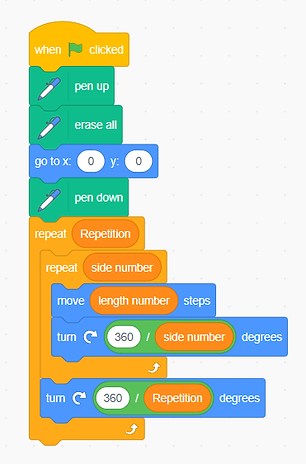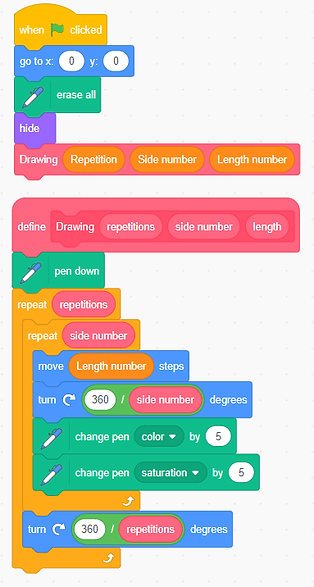top of page

# Rotational Symmetry

Lesson Overview

In this lesson, students will learn how to use programming to create rotational symmetry shapes made by rotating different regular polygons. To finish the programming task, they need to apply the properties of rotation, the definition and properties of regular polygons, and the relationship between interior and exterior angles to write programmes that can automatically draw rotational symmetry figures. The problem-solving process will motivate students to master how to calculate the degrees of the interior and exterior angles of a regular polygon, as well as several important CT concepts and practices (e.g., loops, subroutines, decomposition).

### Prior Knowledge

• Mathematics: definition of a regular polygon; properties of rotation

• Scratch: know how to move and turn the direction of the sprite, able to use pen function to draw

### Learning Objectives

• To acquire the mathematics concept of rotational symmetry and the properties of regular polygons.

• To know how to create rotational symmetry shapes by programming:

• To use nested loop blocks correctly according to the rules for generating rotational symmetry shapes

• To coordinate multiple parameters simultaneously, i.e., side number, side length, and repetition number.

• To experience the meaning of automation and practice of decomposition.

### Mathematics & CT Outcomes

1. Mathematics: properties of polygons, the relationship between interior angles and exterior angles in a polygon, the concept of rotational symmetry

2. CT Skills: loops (repeat XX); subroutines (create my blocks); automation (draw different rotational symmetry shapes automatically); decomposition (break down the graphing process into two steps)

### Teaching Resources

The Desmos Activity used in this Task.

## Lesson Details

We can create beautiful geometric shapes by rotating different polygons!Watch the video to see how they are formed!

Based on the requirements shown in the video, try to choose some code to create a special rotational symmetry!

Click Remix: https://scratch.mit.edu/projects/556173945/

### Guiding Questions

1. What do the three variables shown in the video represent?

2. How do you break down the problem? What is the first step? second step?

3. What will do you in the program to enhance the drawing?

4. Is there any other method to express rotational symmetry in Scratch?

A sample code. Click the image to view the program

#### Students' WorkIn both works, students have broken down the problem into two steps:

(1) first use the [repeat side number] loop block to draw the regular polygon to be rotated [move length number steps, turn 360/side number degrees];

(2) then the angle of each rotation is calculated based on the number of rotations, and the target number of rotations is repeated. As a result, students will gain an in-depth understanding of the relationship between the degree of interior and exterior angles of a regular polygon and the properties of rotation during the problem-solving process.#### Possible Challenges and Misconceptions

(1) Difficulty in drawing regular polygons, i.e., not knowing how to determine the rotation direction and how to calculate the degree of each rotation due to not acquiring the relationship between the interior and exterior angles.

(2) Difficulty in drawing rotated regular polygons, i.e., not being able to use nested loop blocks correctly due to not understanding the rules for forming rotational symmetry shapes.

(3) Easy to ignore examination of invalid cases, i.e., number of sides less than 3, number of rotations less than 2.

## Acknowledgement

The author would like to thank Hin Cheung LAW for designing this lesson and appreciate all the anonymous teachers and students who participated in this research.

bottom of page